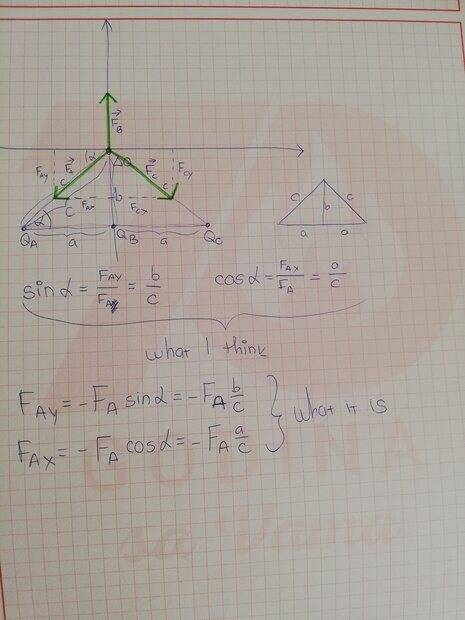# Where did the minus sign come from?

• Edwardy
In summary: Welcome!Could you post the whole problem as assigned?In summary, the problem asks for a presentation of Fay and Fax using Fa and cos(a) and sin(a). The problem appears to be missing a minus, which is needed for the equation cos(a)=-sin(a).

#### Edwardy

Homework Statement
I need to present Fay and Fax using Fa and cos(a) and sin(a). I seem to be missing a minus, why?
Relevant Equations
NoneEdwardy said:
Homework Statement:: I need to present Fay and Fax using Fa and cos(a) and sin(a). I seem to be missing a minus, why?
Relevant Equations:: None

View attachment 315305
There are two ways to think of this.
1) The vector ##F_A## is in the 3rd Quadrant. So both of its vector components will point in the negative directions, thus ##F_{Ax}## and ##F_{Ay}## will be negative.

2) Again, the vector ##F_A## is in the 3rd Quadrant. So the angle it makes with the +x axis is actually ## \theta = 180 + \alpha##. (Or, equivalently, ##\theta = 360 - \alpha ##.) In the 3rd Quadrant ##sin( \theta )## and ##cos( \theta )## are negative.

-Dan

•Edwardy
topsquark said:
There are two ways to think of this.
1) The vector ##F_A## is in the 3rd Quadrant. So both of its vector components will point in the negative directions, thus ##F_{Ax}## and ##F_{Ay}## will be negative.

2) Again, the vector ##F_A## is in the 3rd Quadrant. So the angle it makes with the +x axis is actually ## \theta = 180 + \alpha##. (Or, equivalently, ##\theta = 360 - \alpha ##.) In the 3rd Quadrant ##sin( \theta )## and ##cos( \theta )## are negative.

-Dan
Thank you,
I have one more question though. From my understanding, we are not thinking of Fa as a vector here (hence the arrow above it is missing), so why wouldn't Fa be negative itself? It would have both negative coordinates?

Edwardy said:
Thank you,
I have one more question though. From my understanding, we are not thinking of Fa as a vector here (hence the arrow above it is missing), so why wouldn't Fa be negative itself? It would have both negative coordinates?
I have left the vector notation off here (it messes up the LaTeX for some reason.) The vector ##F_A## has vector components ##F_{Ax}## and ##F_{Ay}##. These are vectors so they have magnitude and direction. The magnitudes of the vectors ##\mid F_A \mid = F_A##, ## \mid F_{Ax} \mid = F_{Ax}##, ## \mid F_{Ay} \mid = F_{Ay}## are just numbers, taken to be positive by convention. The x component of ##F_A## is ##F_{Ax} ( - \hat{i} )## where ##\hat{i}## is the unit vector in the +x direction. The negative is attached to the unit vector direction, not the magnitude.

It is simpler in 1D to just call the components of a vector positive and negative, but it's sloppy and not is not clearly covered in Physics classes. Heck, it wasn't covered clearly in my Math classes, either. But the concept is the same... vectors are never positive or negative. Their components can point in positive and negative directions. This distinction is critical when doing vectors in more than 1D.

-Dan

Edwardy said:
Homework Statement:: I need to present Fay and Fax using Fa and cos(a) and sin(a). I seem to be missing a minus, why?
Relevant Equations:: None
Welcome!
Could you post the whole problem as assigned?
Thank you.

To your original question: when you want a magnitude write it as such. Then there is absolutely no ambiguity $$\vec r=x\hat x + y\hat y$$ where x and y are (signed) numbers. If you wish they can be represented as $$y=|r|sin\theta,~~x=|r|cos\theta$$ and $$\hat x=\hat i,~~\hat y=\hat j$$ In one dimension y=0 always and the x hat is usually omitted.
Not rocket science (oh, wait...)

•topsquark